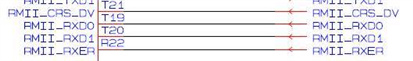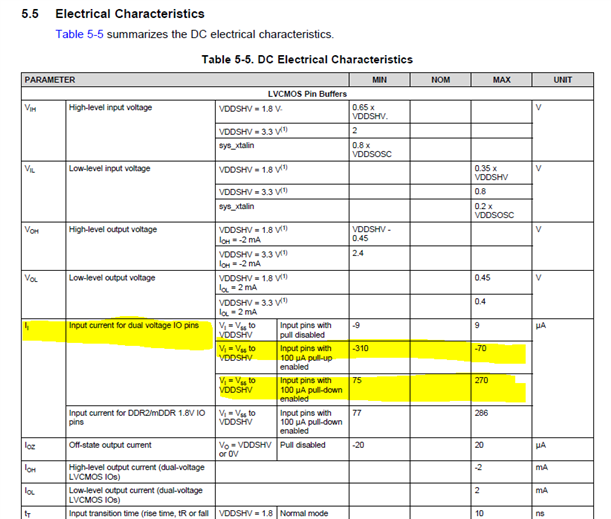If you have a related question, please click the "Ask a related question" button in the top right corner. The newly created question will be automatically linked to this question.

# AM3505: Internal pull-up/down register value

Part Number: AM3505

Hi Team,

The customer is using AM3505AZER with PHY and wants to know the internal pull-up/down register value which is including the variation

There is II : Input current for dual voltage IO pins specification in the data sheet as below. It seems the register is current source. In this case, can we get it by calculation? How to calculate it?

Is the following calculation a correct? I’m not sure the description that Input pins with 100 μA pull-up enable in the data sheet.

• Pull- up
• VDDSHV = 3.3V, Pull-up : 3.3/310=10.6Kohms(Min) 3.3/70=47.1Kohms(Max)
• VDDSHV = 1.8V, Pull-up : 1.8/310=5.8Kohms(Min) 1.8/70=25.7Kohms(Max)
• Pull- down
• VDDSHV = 3.3V, Pull-down : 3.3/270=12.2Kohms(Min) 3.3/75=44.0Kohms(Max)
• VDDSHV = 1.8V, Pull-down : 1.8/270=6.7Kohms(Min) 1.8/75=24.0Kohms(Max)

・RMII_CRS_DV（T21）

・RMII_RXD0（T19）

・RMII_RXD1（T20）

・RMII_RXER（R22）Thanks and Best regards,

Kuerbis

• I assume your usage of "register" in the title of this post along with two instances in the text was meant to be resistor.

The internal pull resistors implemented on the IOs are not linear resistors. The min /max current defined in the electrical characteristics table assumes the IO has the full IO supply potential applied. For example, the min/max pull-up current is defined with an IO voltage potential equal to VSS and the min/max pull-down current is defined with an IO voltage potential equal to respective IO supply voltage. Resistance of the internal pulls are reduced significantly as the voltage potential applied across the pull is decreased. Your calculation will provide an approximate range for the internal pull resistance, but the result is only valid when the IO is at 0V when pulled high and 1.8/3.3V when pulled low.

The internal pulls are primarily provided to hold a unconnected package terminal to a valid logic state.

We recommend external pull resistors if you need to maintain a known logic state on any IO that has a signal trace connected.

Regards,
Paul